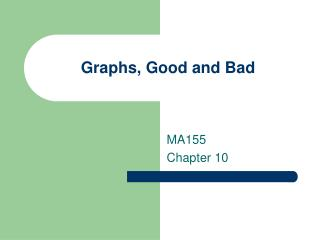Download Presentation# Graphs, Good and Bad - PowerPoint PPT PresentationDownload Presentation- - - - - - - - - - - - - - - - - - - - - - - - - - - E N D - - - - - - - - - - - - - - - - - - - - - - - - - - -
##### Presentation Transcript

1. Graphs, Good and Bad MA155 Chapter 10

2. Organizing Data • The first step in data analysis is to display the data in a graph. • Look first for the overall pattern in the data, and then for striking exceptions to that pattern. • After looking at the graphs, you can use well-chosen numerical descriptions.

3. Describing Distributions • Distributions • The distribution of a variable tells us what value the variable takes and how often each value occurs.

4. Data Tables • Clearly Labeled • Units are stated • Source of Data

5. Eye Color of Students

6. Pie Charts • A whole is divided into parts • Steps • Draw a circle • Make wedges that are representative of the proportion • The parts do make a whole

7. Pie Graph

8. Bar Graph • The height of each bar shows the proportion • It clearly shows the results • They are easy to make

9. Bar Graph

10. Categorical Variable Places an individual into one of several groups or categories Quantitative Variable Takes numerical values for which arithmetic operations make sense Types of Variables

11. Categorical Examples • Race • Sex • Employment Status • Hair Color • Type of Car

12. Quantitative Examples • Measurements made on scales of equal units • Examples • Height in inches • Reaction time in seconds • Temperature in degrees Celsius

13. Remember • Not all numbers carry the same information • Luke is a skateboarder. • Luke finished first at the 2000 Gravity Games • Luke scored a 92.60 on the street course. • The scale of measurement depends on the measuring process, not on the property measured.

14. Displaying Distributions • To display the distribution of a variable measured in a nominal scale, use a pie chart or a bar graph • Pie charts emphasize how individual counts or percents are related to the whole • Bar graphs emphasize how quantities compare with one another. • Use pie charts and bar graphs when displaying the distribution of categorical variables.

15. Pictograms Source: http://www.usatoday.com

16. Line Graphs • Show the behavior of a variable over time • Horizontal Axis Time • Vertical Axis Scale of Variable

17. Scales • The scales of a line graph can cause a graph to be misleading

18. Making Good Graphs • Must be clearly labeled • Units stated • Source of Data • Make the data stand out!

19. Homework • Read Chapter 11 • Suggested Problems: • 10.1, 10.3, 10.9, 10.13, 10.17, 10.27